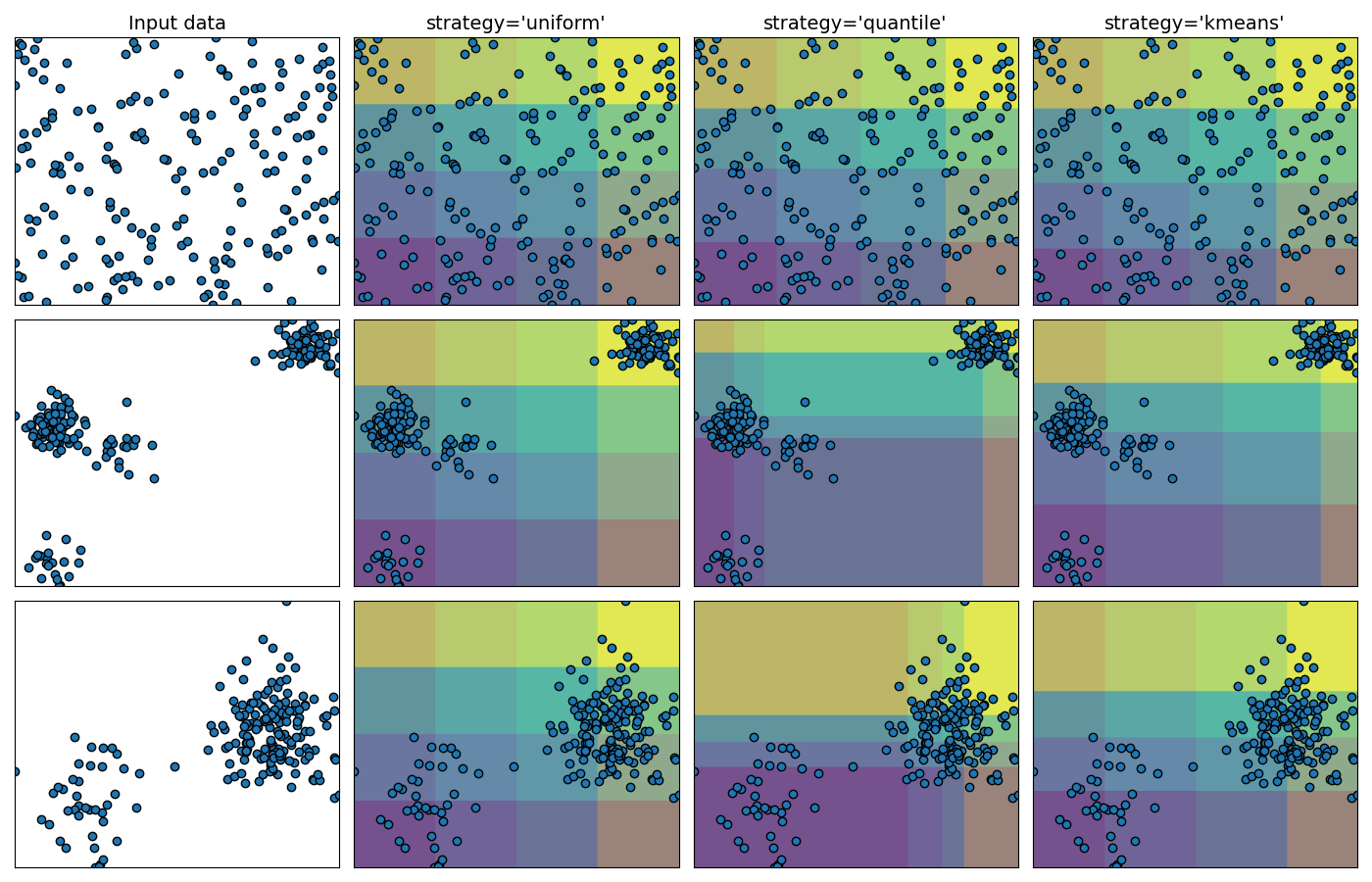# Demonstrating the different strategies of KBinsDiscretizer¶

This example presents the different strategies implemented in KBinsDiscretizer:

• ‘uniform’: The discretization is uniform in each feature, which means that the bin widths are constant in each dimension.

• quantile’: The discretization is done on the quantiled values, which means that each bin has approximately the same number of samples.

• ‘kmeans’: The discretization is based on the centroids of a KMeans clustering procedure.

The plot shows the regions where the discretized encoding is constant.# Author: Tom Dupré la Tour

import numpy as np
import matplotlib.pyplot as plt

from sklearn.preprocessing import KBinsDiscretizer
from sklearn.datasets import make_blobs

print(__doc__)

strategies = ['uniform', 'quantile', 'kmeans']

n_samples = 200
centers_0 = np.array([[0, 0], [0, 5], [2, 4], [8, 8]])
centers_1 = np.array([[0, 0], [3, 1]])

# construct the datasets
random_state = 42
X_list = [
np.random.RandomState(random_state).uniform(-3, 3, size=(n_samples, 2)),
make_blobs(n_samples=[n_samples // 10, n_samples * 4 // 10,
n_samples // 10, n_samples * 4 // 10],
cluster_std=0.5, centers=centers_0,
random_state=random_state),
make_blobs(n_samples=[n_samples // 5, n_samples * 4 // 5],
cluster_std=0.5, centers=centers_1,
random_state=random_state),
]

figure = plt.figure(figsize=(14, 9))
i = 1
for ds_cnt, X in enumerate(X_list):

ax = plt.subplot(len(X_list), len(strategies) + 1, i)
ax.scatter(X[:, 0], X[:, 1], edgecolors='k')
if ds_cnt == 0:
ax.set_title("Input data", size=14)

xx, yy = np.meshgrid(
np.linspace(X[:, 0].min(), X[:, 0].max(), 300),
np.linspace(X[:, 1].min(), X[:, 1].max(), 300))
grid = np.c_[xx.ravel(), yy.ravel()]

ax.set_xlim(xx.min(), xx.max())
ax.set_ylim(yy.min(), yy.max())
ax.set_xticks(())
ax.set_yticks(())

i += 1
# transform the dataset with KBinsDiscretizer
for strategy in strategies:
enc = KBinsDiscretizer(n_bins=4, encode='ordinal', strategy=strategy)
enc.fit(X)
grid_encoded = enc.transform(grid)

ax = plt.subplot(len(X_list), len(strategies) + 1, i)

# horizontal stripes
horizontal = grid_encoded[:, 0].reshape(xx.shape)
ax.contourf(xx, yy, horizontal, alpha=.5)
# vertical stripes
vertical = grid_encoded[:, 1].reshape(xx.shape)
ax.contourf(xx, yy, vertical, alpha=.5)

ax.scatter(X[:, 0], X[:, 1], edgecolors='k')
ax.set_xlim(xx.min(), xx.max())
ax.set_ylim(yy.min(), yy.max())
ax.set_xticks(())
ax.set_yticks(())
if ds_cnt == 0:
ax.set_title("strategy='%s'" % (strategy, ), size=14)

i += 1

plt.tight_layout()
plt.show()


Total running time of the script: ( 0 minutes 0.880 seconds)

Estimated memory usage: 8 MB

Gallery generated by Sphinx-Gallery